# Rule For Determination Of Total Number Of Hybrid Orbitals

## RULE FOR DETERMINATION OF TOTAL NUMBER OF HYBRID ORBITALS

• Detect the central atom along with the peripheral atoms.
• Count the valence electron of the central atom and the peripheral atoms.
• Divide the above value by 8. Then the quotient gives the number of σ bonds and the remainder gives the non-bonded electrons. So number of lone pair =non bonded electrons/ 2.
• The number of σ bonds and the lone pair gives the total number of hybrid orbitals.

### An example will make this method clear

SF4  Central atom S

Peripheral atom F

∴ Total number of valence electrons = 6+ (4 ×7) = 34

Now 8) 34 (4

32/2

∴ Number of hybrid orbital = 4σ bonds + 1 lone pair

So 5 hybrid orbitals are necessary and hybridisation mode is sp3d and it is trigonal bipyramidal (TBP).

Both the structures are TBP. But the lone pair is placed in different position. In B it is placed in equatorial position and in A it is in axial.

Now when a lone pair is in equatorial position the repulsion are minimized. So structure (B) is correct.

Note: Whenever there are lone pairs in TBP geometry they should be placed in equatorial position so that repulsion is minimum.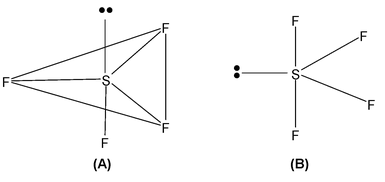1. NCl3  Total valence electrons = 26  Requirement  = 3 σ bonds + 1 lone pair  Hybridsation = sp3 Shape  = pyramidal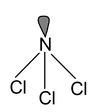2. BBr3 Total valence electron  = 24 Requirement  = 3σ bonds  Hybridisation = sp2 Shape  = planar trigonal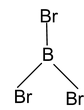3.  SiCl4 Total valence electrons = 32 Requirement  = 4σ bonds  Hybridisation =  sp3 Shape  = Tetrahedral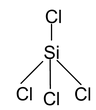4. CI4 Total valence electron = 32 Requirements = 4 σ bonds  Hybridisation = sp3 Shape = Tetrahedral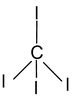5. SF6 Total valence electrons  = 48 Requirement = 6 σ bonds  hybridisation = sp3d2 shape  = octahedral / square bipyramidal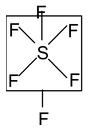6. BeF2 Total valence electrons : 16 Requirement : 2 σ bonds  Hybridisation : sp Shape : Linear F – Be – F 7. ClF3 Total valence electrons : 28 Requirement : 3 σ bonds + 2 lone pairs  Hybridisation : sp3d Shape : T – shaped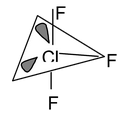We have already discussed that whenever there are lone pairs they should be placed in equatorial positions. Now a question that may come to your mind that though the hybridisation is sp3d, so the shape should be T.B.P. But when all the bonds are present the actual shape is TBP. But when instead of bond there are lone pairs in TBP the actual geometry is determined by the bonds not by the lone pairs. Here in ClF3 the bond present (2 in axial and 1 in equatorial) gives the impression of T shape.

 8. PF5  Total valence electrons : 40 Requirement : 5 σ bonds Hybridisation : sp3d Shape :  Trigonal bipyramidal (TBP)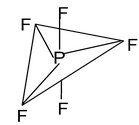9. XeF4 Total valence electrons : 36 Requirement:4σ bonds+ 2 lone pairs  Hybridisation : sp3d Shape :  Square planar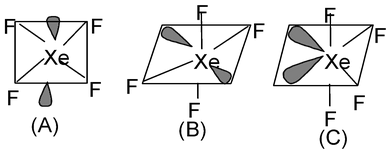Now three arrangements are possible out of which A and B are same. A and B can be inter converted by simple rotation of molecule. The basic difference of (B) and (C) is that in (B) the lone pair is present in the anti positions which minimize the repulsion which is not possible in structure (C) where the lone pairs are adjacent. So in a octahedral structure the lone pairs must be placed at the anti positions to minimize repulsion. So both structure (A) and (B) are correct.

 10. XeF2 Total valence electrons : 22  Requirements : 2σ bonds + 3 lone pairs  Hybridisation : sp3d Shape : Linear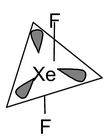[ l.p. are present in equatorial position and ultimate shape  is due to the bonds that are formed]

 11. PF2Br3 Total valence electrons : 40 Requirements : 5 σ bonds  Hybridisation : sp3d Shape : trigonal bipyramidal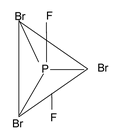Here we see that fluorine is placed in axial position whereas bromine is placed in equatorial position. It is the more electronegative element that is placed in axial position and less electronegative element is placed in equatorial position. Fluorine, being more electronegative pulls away bonded electron towards itself more than that is done by bromine atom which results in decrease in bp – bp repulsion and hence it is placed in axial position.

In this context it can also be noted that in T.B.P. shape the bond lengths are not same. The equatorial bonds are smaller than axial bonds. But in square bipyramidal shape, all bond lengths are same.

 12.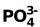Total valence electrons : 32  Requirement : 4 σ bonds  Hybridisation : sp3 Shape : tetrahedral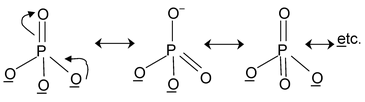Here all the structures drawn are resonating structures with O resonating with double bonded oxygen.

 13. NO2– Total valence electron: 18  Requirement : 2σ bonds + 1 lone pair Hybridisation: sp2 Shape: angular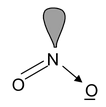14. CO32–  Total valence electrons : 24

Requirement = 3 σ bonds

Hybrdisation = sp2

Shape: planar trigonal

But C has 4 valence electrons of these 3 form σ bonds ∴ the rest will form a π bond.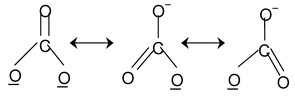In the structure one bond is a double bond and the other 2 are single. The position of the double bonds keeps changing in the figure. Since peripheral atoms are isovalent, so contribution of the resonant structures are equal. Thus it is seen that none of the bonds are actually single or double. The actual state is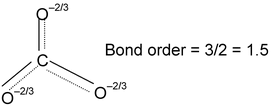15. CO2 Total valence electrons : 16 Requirement: 2σ bonds  Hybridisation: sp Shape: linear O = C = O 16.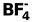Total  valence electrons = 32 Requirement= 4 σ bonds  Hybridisation: sp3 Shape: Tetrahedral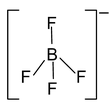17.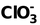Total valence electron = 26 Requirement = 3 σ bond + 1 lone pair  Hybridisation: sp3 Shape: pyramidal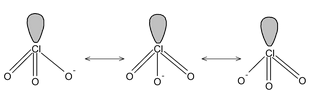18. XeO2F2 Total valence electrons : 34 Requirement: 4 σ bonds +1 lone pair Hybridisation : sp3d Shape: Distorted TBP (sea-saw geometry)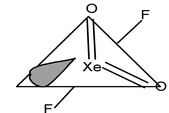19. XeO3 Total valence electrons : 26 Requirement: 3 σ bonds + 1 lone  pair Hybridisation: sp3 Shape: Pyramidal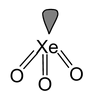20. XeOF4 Total valence electrons : 42 Requirement: 5 σ bonds + 1 lone pair Hybridisation: sp3d2 Shape: square pyramidal.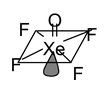### Maximum Covalency:

 Elements which have vacant d-orbital can expand their octet by transferring electrons, which arise after unpairing, to these vacant d-orbital e.g. in sulphur.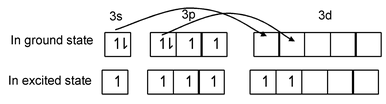In excited state sulphur has six unpaired electrons and shows a valency of six e.g. in SF6. Thus an element can show a maximum covalency equal to its group number e.g. chlorine shows maximum covalency of seven.